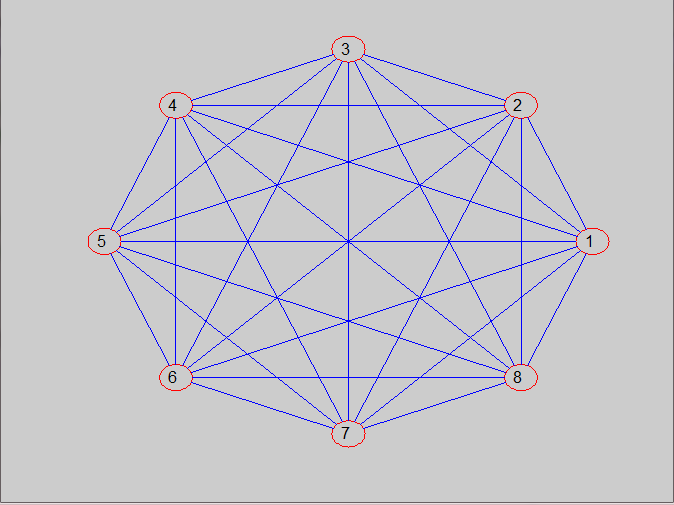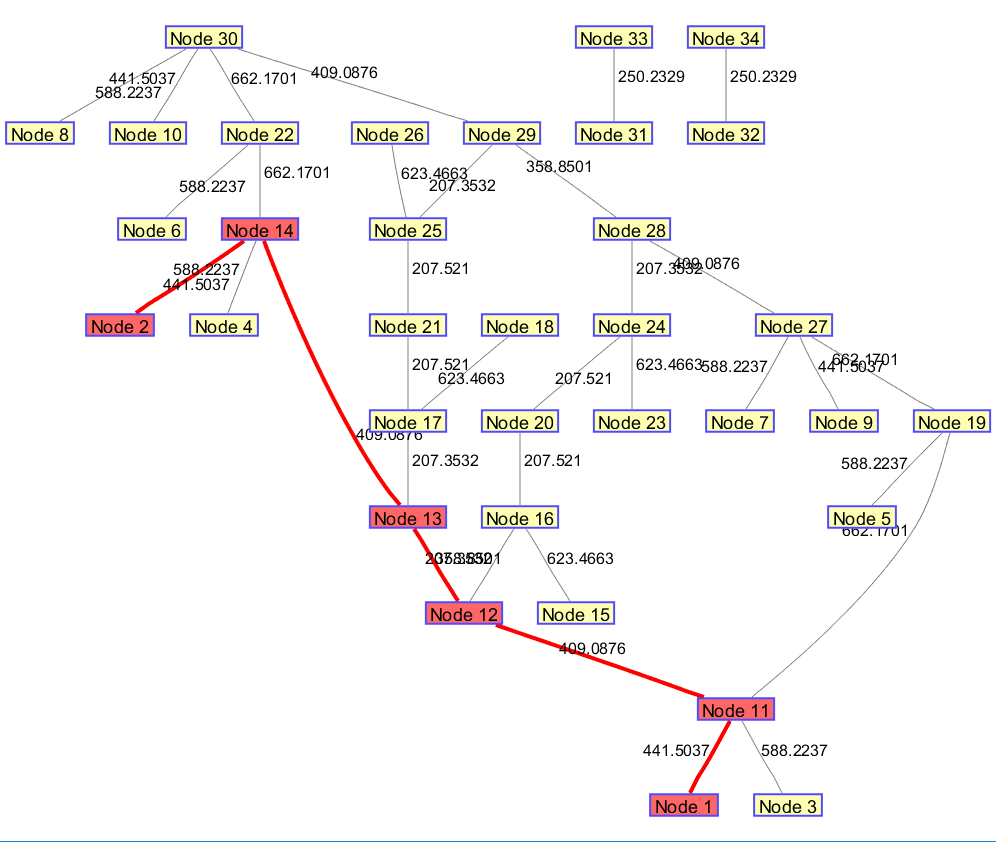• 根据网络邻接矩阵画出有向图或无向图 可用于交通、电能等网络的可视化MATLAB
• %第二个输入为控制量，0表示无向图，1表示有向图。默认值为0r_size=size(rel);%获取矩阵大小if nargin<2 %如果参数小于2，默认无向图control=0;endif r_size(1)~=r_size(2)disp('Wrong Input! The input mu...

function graph(rel,control)

%由邻接矩阵画图

%输入为邻接矩阵，必须为方阵；

%第二个输入为控制量，0表示无向图，1表示有向图。默认值为0

r_size=size(rel);%获取矩阵大小

if nargin<2 %如果参数小于2，默认无向图

control=0;

end

if r_size(1)~=r_size(2)

disp('Wrong Input! The input must be a square matrix!');%输入为邻接矩阵，必须为方阵

return;

end

len=r_size(2);

!echo len;

disp(len);

rho=10;%限制图尺寸的大小

r=1/1.05^len;%点的半径

theta=0:(2*pi/len):2*pi*(1-1/len);%以0开始，步长2*pi/len，结束2*pi*(1-1/len)，相当于有len个点

[pointx,pointy]=pol2cart(theta',rho);

theta=0:pi/36:2*pi;%73个点

!echo theta:

disp(theta);

[tempx,tempy]=pol2cart(theta',r);%73个坐标

point=[pointx,pointy];%6个坐标点

!echo point;

disp(point);

hold on

for i=1:len

temp=[tempx,tempy]+[point(i,1)*ones(length(tempx),1),point(i,2)*ones(length(tempx),1)];%73行1列的1矩阵与point每个坐标相乘

plot(temp(:,1),temp(:,2),'r');%plot(x,y),画出圆圈

%plot(point(:,1),point(:,2),'r');%plot(x,y)

text(point(i,1)-0.3,point(i,2),num2str(i));

%画点

end

for i=1:len

for j=1:len

if rel(i,j)%如果邻接矩阵rel中该点是1，代表相连

%连接有关系的点

end

end

end

set(gca,'XLim',[-rho-r,rho+r],'YLim',[-rho-r,rho+r]);

axis off

%%

%连接两点

temp=point2-point1;

if (~temp(1))&&(~temp(2))

return;

%不画子回路；

end

theta=cart2pol(temp(1),temp(2));

[point1_x,point1_y]=pol2cart(theta,r);

point_1=[point1_x,point1_y]+point1;

[point2_x,point2_y]=pol2cart(theta+(2*(theta

在命令行：

A=ones(8);

graph(8)展开全文• 这里有一点一定要注意，因为为无向图，因此邻接矩阵一定要是关于对角线对称的，即Aij=Aji（且对角线上元素Aii=0），两点之间相互无向连接，有向图可以不...%% Matlab 无向图 % 生成数据,A和邻接矩阵的形势相似 A = magi
• 今天小编就为大家分享一篇关于判断一个无向图是否为连通图的方法，小编觉得内容挺不错的，现在分享给大家，具有很好的参考价值，需要的朋友一起跟随小编来看看吧
• matlab无向图代码FI-GRL：通过投影成本保留进行快速归纳图表示学习 作者：费飞（） 概述 这是一种使用随机投影的图嵌入算法，可在理论上保证快速，准确地嵌入。 要求 该算法在Matlab中实现。 验证了2016_a的matlab...
• 这是MATLAB工具箱函数生成的一个带权无向图，图中红色的节点和线表示Node1与Node2之间的最短通路。 使用graphshortestpath函数，可以输出： ①. 图中任意两个节点之间的最短距离 ②. 任意两个节点之间的最短路径...

这是MATLAB工具箱函数生成的一个带权无向图，图中红色的节点和线表示Node1与Node2之间的最短通路。

使用graphshortestpath函数，可以输出：

①. 图中任意两个节点之间的最短距离

②. 任意两个节点之间的最短路径：此处为1→11→12→13→14→2   （数字为图中节点编号）附上代码：

start=data(:,1);  %起点编号
endot=data(:,2);   %终点编号
weight=data(:,3);  %连接起点和终点的边权重
graphsize=max(max(start),max(endpt));

%更新距离矩阵
DG = sparse(start,endpt,weight,graphsize,graphsize)%求稀疏矩阵
g =tril(DG+DG')%取矩阵和转置矩阵和的下三角矩阵
%这一步的目的是要把稀疏矩阵化为无向

%建立无向图
p=biograph(g,[],'ShowArrows','off','ShowWeights','on');
h=view(p);%显示各个路径权值

% 求节点1到节点2的最短路径
[Dist,Path]=graphshortestpath(g,1,2,'Directed',false,'Method','Dijkstra')

% 将最短路径的结点以红色显示
set(h.Nodes(Path),'Color',[1 0.4 0.4]);
% 将最短路径的弧以红色显示
edges=getedgesbynodeid(h,get(h.Nodes(Path),'ID'),get(h.Nodes(Path),'ID'));
set(edges,'LineColor',[1 0 0]);
set(edges,'LineWidth',2.0);
展开全文MATLAB
• 无向图中最大团问题的matlab代码，利用的算法是回溯法，代码包含MCP函数、测试代码和根据邻接矩阵画无向图函数
• matlab 绘制有向图、无向图、有权有向图、有权无向图1、Matlab作无权无向图2、Matlab作有权无向图3、Matlab作无权有向图4、Matlab作有权有向图 1、Matlab作无权无向图 % 函数graph(s,t)：可在 s 和 t 中的对应节点...

1、Matlab作无权无向图

% 函数graph(s,t)：可在 s 和 t 中的对应节点之间创建边，并生成一个图
% s 和 t 都必须具有相同的元素数；这些节点必须都是从1开始的正整数，或都是字符串元胞数组。
s1 = [1,2,3,4];
t1 = [2,3,1,1];
G1 = graph(s1, t1);
plot(G1)
% 注意哦，编号最好是从1开始连续编号，不要自己随便定义编号

效果图：还可以用字体代表节点：

% 注意字符串元胞数组是用大括号包起来的哦
s2 = {'学校','电影院','网吧','酒店'};
t2 = {'电影院','酒店','酒店','KTV'};
G2 = graph(s2, t2);
plot(G2, 'linewidth', 2)  % 设置线的宽度
% 下面的命令是在画图后不显示坐标
set( gca, 'XTick', [], 'YTick', [] );

效果：2、Matlab作有权无向图

% 函数graph(s,t,w)：可在 s 和 t 中的对应节点之间以w的权重创建边，并生成一个图
s = [1,2,3,4];
t = [2,3,1,1];
w = [3,8,9,2];
G = graph(s, t, w);
plot(G, 'EdgeLabel', G.Edges.Weight, 'linewidth', 2)
set( gca, 'XTick', [], 'YTick', [] );3、Matlab作无权有向图

% 无权图 digraph(s,t)
s = [1,2,3,4,1];
t = [2,3,1,1,4];
G = digraph(s, t);
plot(G)
set( gca, 'XTick', [], 'YTick', [] );4、Matlab作有权有向图

s = [1,2,3,4];
t = [2,3,1,1];
w = [3,8,9,2];
G = digraph(s, t, w);
plot(G, 'EdgeLabel', G.Edges.Weight, 'linewidth', 2)
set( gca, 'XTick', [], 'YTick', [] );5、查找有权图的最短路径

s = [9 9 1 1 2 2 2 7 7 6 6  5  5 4];
t = [1 7 7 2 8 3 5 8 6 8 5  3  4 3];
w = [4 8 3 8 2 7 4 1 6 6 2 14 10 9];
G = graph(s,t,w);
plot(G, 'EdgeLabel', G.Edges.Weight, 'linewidth', 2)
set( gca, 'XTick', [], 'YTick', [] );
[P,d] = shortestpath(G, 9, 4)  %注意：该函数matlab2015b之后才有哦 P里面存储的就是最短路径上面的节点

% 在图中高亮我们的最短路径
myplot = plot(G, 'EdgeLabel', G.Edges.Weight, 'linewidth', 2);  %首先将图赋给一个变量
highlight(myplot, P, 'EdgeColor', 'r')   %对这个变量即我们刚刚绘制的图形进行高亮处理（给边加上r红色）

% 求出任意两点的最短路径矩阵
D = distances(G)   %注意：该函数matlab2015b之后才有哦
D(1,2)  % 1 -> 2的最短路径
D(9,4)  % 9 -> 4的最短路径

% 找出给定范围内的所有点  nearest(G,s,d)
% 返回图形 G 中与节点 s 的距离在 d 之内的所有节点
[nodeIDs,dist] = nearest(G, 2, 10)   %注意：该函数matlab2016a之后才有哦参考资料来源：数学建模清风老师

展开全文• 函数旨在实现 Ricardo Simões 在... 此代码已从算法中修改，以便获得随机连接的无向图的任意两个节点之间的所有路径，而无需查找循环。 这是我的研究工作的要求，即不需要循环。 所以 APAC 算法被修改为只计算路径。matlab
• matlab求无向图的最短路径

千次阅读 2021-04-11 21:50:33
无向图的连通分支，或有向图的强（弱）连通分支 graphisdag 测试有向图是否含有圈，不含圈返回1，否则返回0 graphisomorphism 确定两个图是否同构，同构返回1，否则返回0 graphisspantree 确定一个图是否生

1 matlab图论工具箱的函数

matlab图论工具箱主要用于求最短路径，最小生成树和最大流。
其常见命令如下表：

函数名功能
graphallshortestpaths求图中所有顶点对之间的最短距离
graphconncomp找无向图的连通分支，或有向图的强（弱）连通分支
graphisdag测试有向图是否含有圈，不含圈返回1，否则返回0
graphisomorphism确定两个图是否同构，同构返回1，否则返回0
graphisspantree确定一个图是否生成树，是返回1，否则返回0
graphmaxflow计算有向图的最大流
graphminspantree在图中找最小生成树
graphpred2path把前驱顶点序列变成路径的顶点序列
graphshortestpath求图中指定的一对顶点间的最短距离和最短路径
graphtopoorder执行有向无圈图的拓扑排序
graphtraverse求从一顶点出发，所能遍历图中的顶点

2 求无向图中任意两点间的最短路径

图片如下:图中M2_1和M2_2之间的数字2057代表两点之间的距离。matlab中函数graphshortestpath用于求无向图任意两点间的最小距离。根据无向图，需要构造任意相邻两点之间的距离矩阵。
例如：

a(1,2)=2057 ;a(1,3)=2031;

代表点M2_1和点M2_2之间的距离为2057，点M2_1和点M2_3之间的距离为2031

matlab编程实现求图中任意两点之间的距离最小值。

clc;
clear;
close all;
% 计算任意两个之间的最小距离
sum = 0;  %保存距离求和
a(1,2)=2057 ;a(1,3)=2031;   %1和点2之间的距离为2057，点1和点3之间的距离为2031
a(2,3)=1967;  a(2,5)=1042; a(2,6)=1403;
a(3,4)=821;  a(3,5)=1115;
a(4,9)=2281;
a(5,8)=1905;
a(6,7)=1329;
a(7,8)=1666;
a(8,9)=1513;
a = a';  %matlab工具箱要求数据是下三角矩阵
[i,j,v] = find(a);
b = sparse(i,j,v,9,9); %构造稀疏矩阵
[x,y,z] = graphshortestpath(b,1,9,'directed',false);  %函数括号内19分别代表图中第一和第九个顶点，更改数字即可求任意两点的最小距离，x为计算得到的19两点间的最小距离

函数计算结果，x为5133，y为从点1到点9得到的最短距离经过的点，输出为[1 3 4 9]代表经过了第3点和第4点。

展开全文• 该程序根据某些目标函数使用局部搜索优化来找到无向图的近似最佳聚类。 支持许多不同的目标。 有八度和MATLAB的绑定。 形式上，要解决的问题是在图中找到节点的聚类C，以最大程度地减少损失 loss(C) = f(sum_{c ∈ C...
• [MC] = MAXIMALCLIQUES(A,V_STR) 给定图的... 参考：Bron、Coen 和 Kerbosch，Joep，“算法 457：查找无向图的所有集团”，ACM 通信，卷。 16，没有。 9, pp: 575–577, 1973 年 9 月。 参考：Cazals, F. 和 Karande,matlab
• matlab无向图代码 N跳图邻域计算 信息 问题 给定一个图G =（V，E） （有向或无向），计算给定图中节点的N跳邻域 方法 没什么神奇的。 简单的穷举计算。 没有尝试使其平行。 但是，通过使用MATLAB已有的并行化工具...
• 一 概述 其实MATLAB的GUI完全可以使用MATLAB中各种控件的函数来实现，也可以说GUI就是一个figure，本人也是对MATLAB GUI比较感兴趣的一个学习者，在学习MATLAB过程中，搜索了不少资料才了解了用函数形式也就是不依靠...
• 这是用于处理简单图（没有循环或多边的无向图）的广泛函数套件。 它包括三个新类：图、排列、分区。 一些函数需要优化工具箱。 请阅读文档，因为图形对象以一种不寻常的方式定义，这使得将它们传递给函数有效并使...matlab
• MATLAB 函数处理图像实现膨胀腐蚀一、实验目的1、了解二值形态学的基本运算2、掌握二值图像膨胀、腐蚀的基本方法3、编程实现膨胀、腐蚀二、实验要求1、使用imdilate 函数进行图像膨胀，并观察膨胀后图像的变化。...
• 图论--寻找节点间所有路径最近在...在中存在着7个节点，连通情况如下： 首先，想到的就是树中的深度遍历。因为我们需要循着上轮访问的节点，才能确定整个路径。如果仅仅完成没有问题，最关键的在于如何记录下所有...
• Matlab 的函数

2021-04-18 12:00:30
nbsp预备知识　Matlab 的判断与循环函数文件我们已经学了一些函数，现在来看如何自定义函数Matlab 中定义了函数的文件叫做函数文件．函数文件同样以 “.m” 作为后缀名，文件中的第一个命令必须是 function，用于...
• matlab plot绘图函数官方详解

万次阅读 多人点赞 2020-06-28 22:47:24
二维线图函数 语法 plot(X,Y) plot(X,Y,LineSpec) plot(X1,Y1,...,Xn,Yn) plot(X1,Y1,LineSpec1,...,Xn,Yn,LineSpecn) plot(Y) plot(Y,LineSpec) plot(___,Name,Value) plot(ax,___) h = plot(___) ...matlab plot
• 函数“generateSpanningTrees(A)”为由邻接矩阵A描述的连通无向简单生成所有生成树。它是“算法S”在p上的实现。 Donald E. Knuth 所著的“计算机编程艺术”一书第 464 卷，第 4A 卷（组合算法，第 1 部分）。 ...matlab
• matlab图像处理常用函数大全

万次阅读 多人点赞 2018-07-16 16:03:34matlab函数 数字图像处理函数
• 无向图的遍历(BFS+DFS，MATLAB)

万次阅读 多人点赞 2017-08-12 20:46:44
广度优先搜索（也称宽度优先搜索，缩写BFS，以下采用广度来描述）是连通的一种遍历策略。因为它的思想是从一个顶点V0开始，辐射状地优先遍历其周围较广的区域，故得名。  一般可以用它做什么呢？一个最直观经典...数学 dfs bfs 搜索 遍历
• 较全的matlab 命令和函数

千次阅读 2020-12-23 15:20:16
d菱形 -- 虚线 ^ 向上箭头3、可以用subplot(3，3，1)...=================================================================附录1.1 管理用命令函数名 功能描述 函数名 功能描述addpath 增加一条搜索路径 rmpath...
• Matlab学习笔记 绘图函数详解

千次阅读 2014-07-12 20:10:54
下面介绍MATLAB 的两种基本绘图功能：二维平面图形和三维立体图形。 5.1 二维平面图形 5.1.1 基本图形函数 plot 是绘制二维图形的最基本函数，它是针对向量或矩阵的列来绘制曲线的。也就是 说，使用plot 函数...matlab
• 该包包含两个函数，BK_MaxIS 和 BK_MaxClique，它们使用 Bron-Kerbosch 算法分别列出给定无向图的所有最大独立集和最大团。 函数的输入是所需无向图的邻接矩阵 ( ...matlab
• Matlab图论工具箱的应用 Matlab图论工具箱 ... 找无向图的连通分支，或有向图的强弱连通分支 graphisdag 测试有向图是否含有圈，不含圈返回1，否则返回0 graphisomorphismmatlab...

matlab无向图函数matlab 订阅